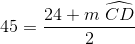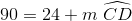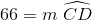# High School Math : How to find the angle for a percentage of a circle

## Example Questions

### Example Question #1 : Circles

A sector comprises 20% of a circle. What is the central angle of the sector?Explanation:

Proporations can be used to solve for the central angle. Letequal the angle of the sector.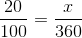Cross mulitply: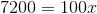Solve for: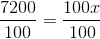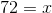### Example Question #2 : Circles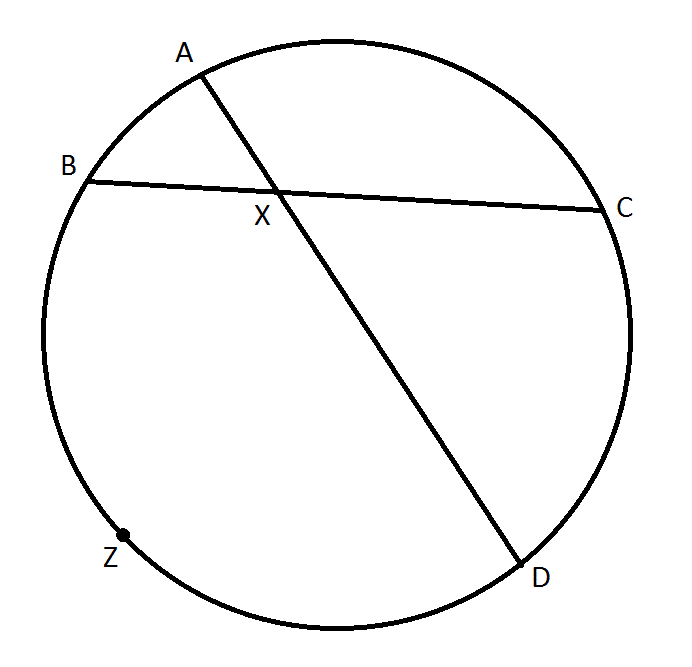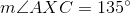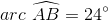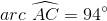Find the degree measure of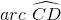.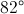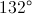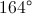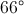Not enough information is given to answer this question.Explanation:

When two chords of a circle intersect, the measure of the angle they form is half the sum of the measures of the arcs they intercept. Therefore,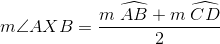Since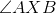and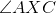form a linear pair,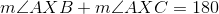, and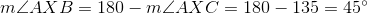.

Substitute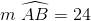and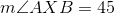into the first equation: